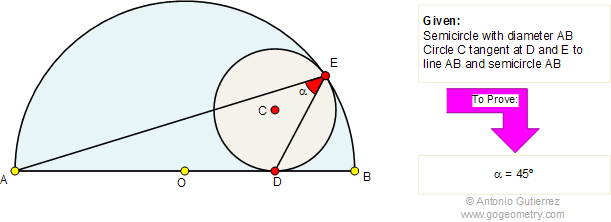Problem 310: Circle Inscribed in a Semicircle, 45 Degrees Angle.
Level: High School, SAT Prep, College geometry.

In the figure below, circle C is inscribed in a semicircle with diameter AB. If D and E are the tangency points, prove that the measure of angle AED is 45 degrees.Sketch and Typography of Problem 310

In the figure below, circle C is inscribed in a semicircle with diameter AB. If D and E are the tangency points, prove that the measure of angle AED is 45 degrees.